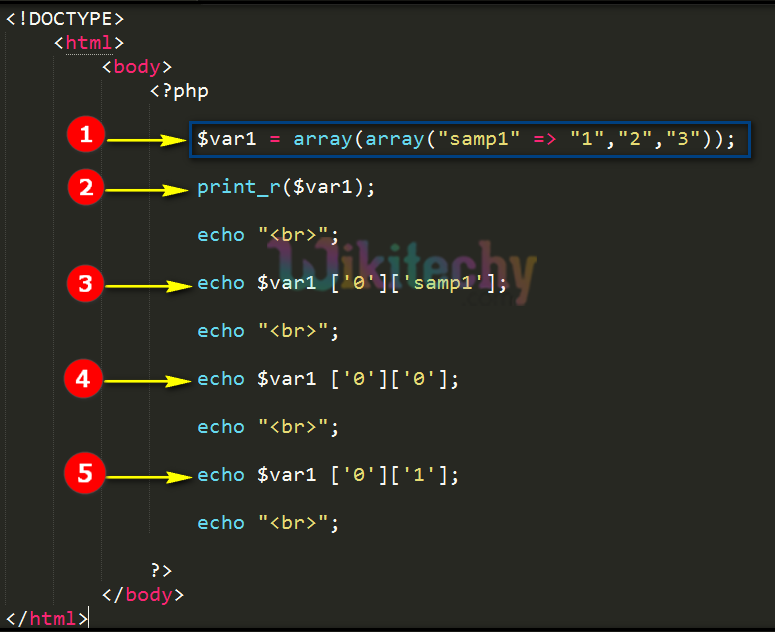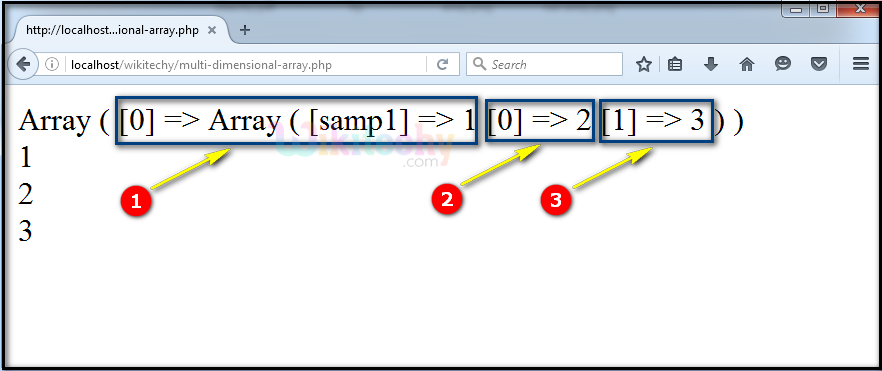# php tutorial - PHP Multidimensional Arrays - php programming - learn php - php code - php script

• In php the multidimensional array contains one or more array in a single array.
• The dimension of an array is defined by number of indices to be selected elements in an array.
• For example, the array contains two indices to be selected elements so, it is called as two dimensional arrays.

## php program Code Explanation :1. In this statement we define the multidimensional array for the variable “\$var1” by using array function and inside the array function we define another array name as ”samp1” and the values are 1,2 and 3.
2. print_r(\$var1); statement prints the php array structure.
3. In this echo statement we print the value of the “\$var1” variable that holds the first row of the php array that will be indexed as (0) and the first column value in this example “samp1” as php array is stored in this index and here we using associative array php function.
4. In this echo statement we print the value of the “\$var1” variable that holds the first row of the array that will be indexed as (0) and the second column value.
5. In this echo statement we print the value of the “\$var1” variable that holds the first row of the php array that will be indexed as (1) and the third column value.

## php coding Sample Output :1. array php structure stores the format with its index value of “samp1” as “1”.
2. Then the display of the index value for “0” as 2.
3. Then the display of the index value for “1” as 3.